# Ap physics 1 Ch 10 Test b systems of Particles & Momentum

 Page 1/2 Date 28.05.2018 Size 134.05 Kb.
1   2
AP Physics 1 Ch 10 Test B

Systems of Particles & Momentum

Name _______________________________ Period ______

*5pts **10pts
 _____1. *Two identical bodies of mass M move with equal speeds v collide in a perfectly elastic collision. The direction of their velocities is illustrated below. The magnitude of the linear momentum of the system right after the collision is? SHOW WORK A) 2 Mv B) Mv C) 4 Mv D)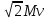E)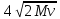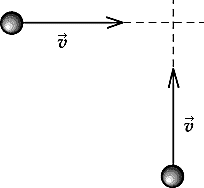_____2. *Two bodies A and B move toward each other with velocities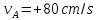and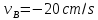. The mass of A is 140 g and the mass of B is 60 g. After a head-on collision the velocity of A is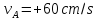. What is the velocity of B after the collision? SHOW WORK

1. + 26.7 cm/s

2. ─ 26.7 cm/s

3. + 44.7 cm/s

4. ─ 44.7 cm/s

5. Not enough information to calculate

 _____3. *If you take the derivative of the kinetic energy of a particle with respect to its velocity, you get A) force. B) momentum. C) acceleration. D) mass. E) potential energy. _____4.*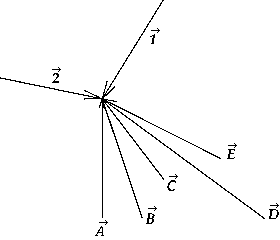Vectors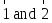are the momentum vectors of two equal masses, m1 and m2. A third equal mass, m3, has a momentum such that when the three masses collide and stick together the combined mass has zero velocity. The vector that best represents the momentum of mass m3 is A)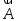B)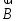C)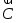D)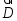E)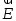_____5.*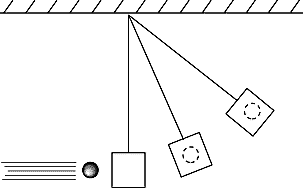The figure shows a ballistic pendulum in three states. The system (considered to be the ball and the pendulum) will move in such a way that A) the kinetic energy is conserved during the collision. B) the linear momentum is conserved after the collision. C) the linear momentum is not conserved during the collision. D) the total mechanical energy is conserved during the collision. E) the total mechanical energy is conserved after the collision.